# Precalculus : Find the Slope of a Line Tangent to a Curve At a Given Point

## Example Questions

### Example Question #1 : Find The Slope Of A Line Tangent To A Curve At A Given Point

Find the slope of the line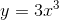at the point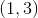.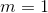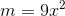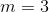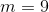Explanation:

First find the slope of the tangent to the line by taking the derivative.

Using the Exponential Rule we get the following,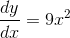.

Then plug 1 into the equation as 1 is the point to find the slope at.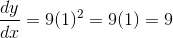.

### Example Question #1 : Find The Slope Of A Line Tangent To A Curve At A Given Point

Find the slope of the following expression at the point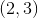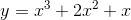.Explanation:

One way of finding the slope at a given point is by finding the derivative. In this case, we can take the derivative of y with respect to x, and plug in the desired value for x.Using the exponential rule we get the following derivative,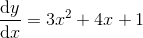.

Plugging in x=2 from the point 2,3 gives us the final slope,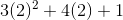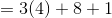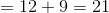Thus our slope at the specific point is.

Note that in this case, using the y coordinate was not necessary.

### Example Question #2 : Find The Slope Of A Line Tangent To A Curve At A Given Point

Find the slope of the tangent line of the function at the given value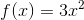at.Explanation:

To find the slope of the tangent line of the function at the given value, evaluate the first derivative for the given.

The first derivative is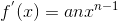and for this function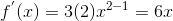and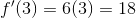So the slope is### Example Question #3 : Find The Slope Of A Line Tangent To A Curve At A Given Point

Find the slope of the tangent line of the function at the given value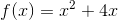at.Explanation:

To find the slope of the tangent line of the function at the given value, evaluate the first derivative for the given value.

The first derivative isand for this function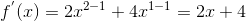and plugging in the specific x value we get,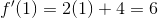So the slope is.

### Example Question #1 : Find The Slope Of A Line Tangent To A Curve At A Given Point

Consider the function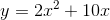.  What is the slope of the line tangent to the graph at the point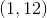?Explanation:

Calculate the derivative ofby using the derivative rules.  The derivative function determines the slope at any point of the original function.

The derivative is: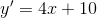With the given point.   Substitute this value to the derivative function to determine the slope at that point.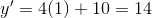The slope of the tangent line that intersects pointis.

### All Precalculus Resources